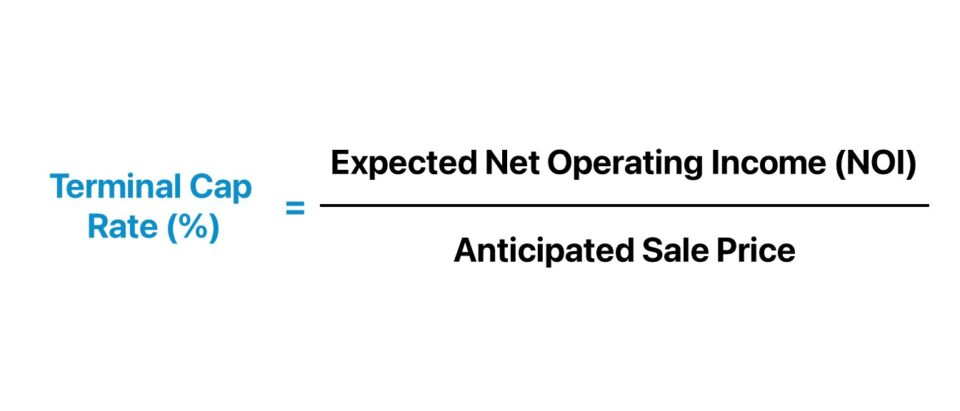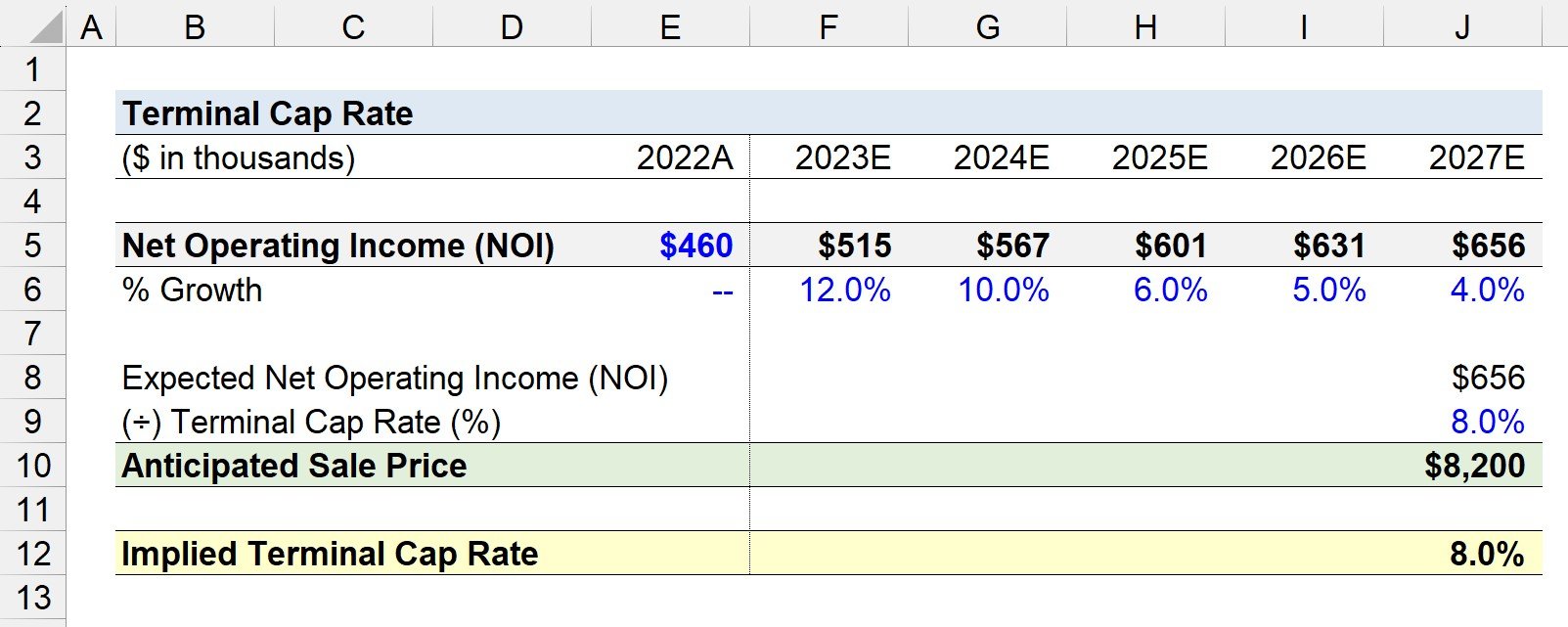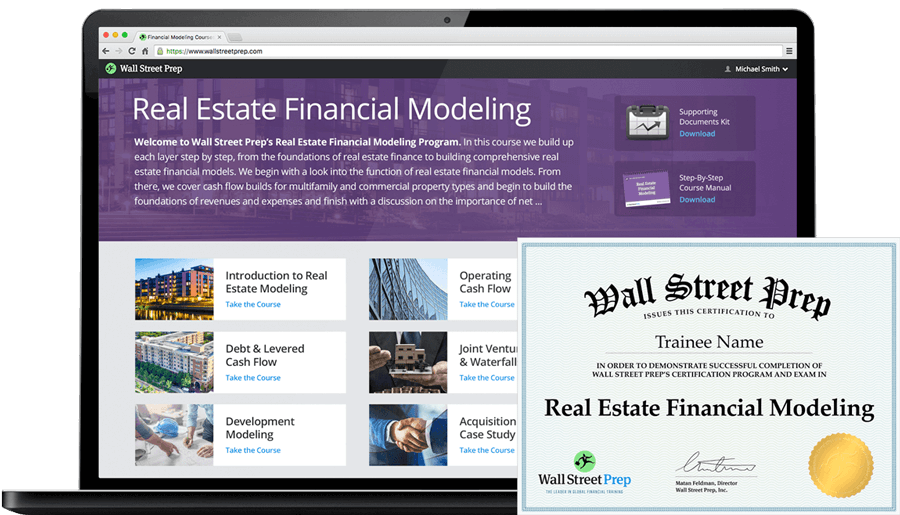Welcome to Wall Street Prep! Use code at checkout for 15% off.# Terminal Cap Rate

Guide to Understanding the Terminal Cap Rate## How to Calculate Terminal Cap Rate?

The terminal cap rate is determined by the prevailing market conditions in the real estate (and credit) markets on the date the seller anticipates exiting the property investment.

The real estate investor can set their own sale price, but the state of the real estate market ultimately decides the exit valuation of the property, i.e. the supply and demand dynamics in the market.

Therefore, a real estate investor must understand the terminal cap rate is merely an estimate, rather than the actual return realized on the date of exit.

The actual cap rate on the date of the official sale – in which ownership of the property changes hands – can deviate substantially from the terminal cap rate computed by the property owner.

The property owner’s bias often inflates the property value, yet the actual determinant of the sale value is due to the market conditions – hence, the importance of timing the exit right.

1. Project Net Operating Income (NOI) → To calculate the terminal cap rate, the first step is to project the property investment’s future net operating income (NOI) on the date of the sale.
2. Calculate Anticipated Sale Price → Using the property’s expected net operating income (NOI), the anticipated sale price can be calculated by multiplying the terminal cap rate by the expected NOI.

One common misconception is that the terminal cap rate is a subjective measure set by the seller, i.e. at random. In fact, the actual sale price is influenced by factors such as current market conditions, trends in the economy (and location), and the outlook on the market by investors on the date of sale.## Terminal Cap Rate Formula

In the formula to calculate the anticipated sale price at exit, the expected net operating income (NOI) of the property on the date of sale is divided by the terminal cap rate.

Anticipated Sale Price = Expected Net Operating Income (NOI) ÷ Terminal Cap Rate

The net operating income (NOI) measures the profitability of a property, net of any direct operating expenses incurred.

Net Operating Income (NOI) = Effective Gross Income (EGI) – Direct Operating Expenses

The effective gross income (EGI) is equal to the sum of a property’s rental income and ancillary income, net of vacancy and credit losses.

By rearranging the formula, we can solve for the terminal cap rate.

Terminal Cap Rate (%) = Expected Net Operating Income (NOI) ÷ Anticipated Sale Price

Therefore, the net operating income (NOI) shares numerous commonalities with EBITDA, and practitioners widely used the two metrics, namely because of their comparability.

Why? Both NOI and EBITDA are capital structure independent metrics, so unaffected by discretionary financing decisions, jurisdiction-dependent income taxes, and accounting methods.

The net operating income (NOI) is computed prior to any debt costs (e.g. principal, interest payments), capital expenditures (Capex), and non-cash add-backs such as depreciation.

## Going-In Cap Rate vs. Terminal Cap Rate: What is the Difference?

The going-in cap rate and the terminal cap rate are variations of the capitalization rate frequently used in the field of real estate investing.

The distinction between the going-in vs. terminal cap rate stems from the context of performing the analysis, i.e. the timing of the calculation.

• Going-In Cap Rate → The going-in cap rate, or “entry cap rate,” is the implied rate of return on a property investment based on the fundamentals of the property and the expected return that the property could potentially generate following the initial purchase.
• Terminal Cap Rate → The numerator in the formula to estimate the going-in cap rate of a portfolio is the expected net operating income (NOI) at exit, whereas the denominator is the projected sale cost.

The formula used to calculate the going-in cap rate, or “entry cap rate”, is as follows.

Going-In Cap Rate = Net Operating Income (NOI) ÷ Purchase Cost

Therefore, the primary distinction between the going-in cap rate and the terminal cap rate is that the former is a method to analyze the potential range of expected returns on the date of initial purchase, while the latter is based on the seller’s assumption of the expected return that could be obtained on the property post-sale.

However, the property owner – irrespective of the fact that it could be inadvertent – might not price the property based on an objective analysis because of his/her own monetary incentives, in which selling the property investment at the maximum valuation results in the seller reaping more profits.

## Terminal Cap Rate Calculator

We’ll now move to a modeling exercise, which you can access by filling out the form below.#### Excel TemplateSubmitting...

## 1. Commercial Real Estate Property Assumptions (CRE)

Suppose a commercial real estate (CRE) investment firm acquired a commercial property for \$4 million at the end of 2022.

Over the course of the latest fiscal year, the commercial property reported a total of \$460k in net operating income (NOI).

• Net Operating Income (NOI), 2022A = \$460,000

However, favorable tailwinds unexpectedly emerged in the market with opportunities for the firm to apply strategic improvements to the property.

In effect, the commercial real estate firm decides to adjust its original forecast to the “upside” case of its model.

By the end of Year 5 – the timing of the planned exit – the real estate firm anticipates the property investment’s net operating income (NOI) to reach \$656k.

• Property Investment Holding Period = 5 Years
• Expected Net Operating Income (NOI) = \$460,000

The implied year-over-year (YoY) growth rates from the end of 2022 to 2027 are as follows.

• 2022 → 2023 = 12.0% YoY
• 2023 → 2024 = 10.0% YoY
• 2024 → 2025 = 6.0% YoY
• 2025 → 2026 = 5.0% YoY
• 2026 → 2027 = 4.0% YoY

## 2. Exit Cap Rate Anticipated Sale Price Calculation Example

Upon performing diligence on the current market – both at the macro level and the specific location of the property – the firm estimates the property’s terminal cap rate, or exit cap rate, to be approximately 8.0% on the date of sale.

• Terminal Cap Rate (%) = 8.0%

The terminal cap rate is determined by analyzing comparable properties and conducting research on the location (and its long-term outlook), which we’ll assume is how the real estate firm arrived at 8.0%.

The real estate firm can compute the anticipated sale price by dividing the exit year net operating income (NOI) by the estimated terminal cap rate.

Upon dividing the property’s net operating income (NOI) of \$480k in Year 5 – fiscal year ending 2027E – by the market cap rate of 8.0%, the anticipated sale price of the property comes out to \$8.2 million.

• Anticipated Sale Price = \$656,000 ÷ 8.0% = \$8,200,000

The property’s terminal cap rate is assumed to be 8.0% in our illustrative example.

• Terminal Cap Rate (%) = 8.0%

If there is a buyer in the market receptive to the pricing – ignoring factors such as inflation, financing costs and transaction costs (i.e. professional fees) – the commercial property could fetch a total sale price of \$6 million.

## 3. Terminal Cap Rate Calculation Example

In conclusion, we will back-solve for the terminal cap rate using the financial data accumulated thus far. To reiterate from earlier, the terminal cap rate is calculated by dividing the expected net operating income (NOI) by the anticipated sale price.

The implied terminal cap rate is 8.0%, which matches our original assumption stated at the start of our quick tutorial.

• Implied Terminal Cap Rate = \$656k ÷ \$8.2 million = 8.0%20+ Hours of Online Video Training

### Master Real Estate Financial Modeling

This program breaks down everything you need to build and interpret real estate finance models. Used at the world's leading real estate private equity firms and academic institutions.

Inline Feedbacks